# Tetris Turns 35

## On June 6, 2019 Tetris turned 35 years old. To celebrate, I wrote my own version and posted it in my GitHub repo. No libraries. No frameworks. Just JavaScript. Those learning JavaScript might find a few lessons here!

Here’s a list of my best web development tutorials.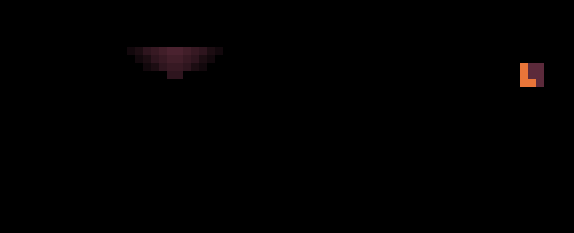Tetris In The Dark I developed to celebrate 35 years of Tetris!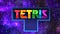The new Tetris logo was recently announced on June 6, 2019, the game’s 35th anniversary of the game. This image was borrowed from the Twin Galaxies article, a neat gaming site, check it out. The Tetris logo is copyright The Tetris Company, LLC with its headquarters Honolulu, HI.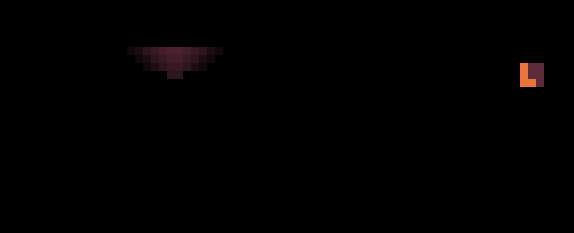Tetris In The Dark.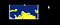First attempt at making Tetris.
`let color = { background: "#5c2a3b",     // background                    wall: "#d83c66",     // walls                   solid: "#49b5ab",     // solid tetromino               tetromino: "#e97539" };   // falling tetromino`

## Complete Tetris Source Code

This tutorial is based on existing Tetris code I wrote a week ago. You can fork the Complete Tetris source code from my GutHub profile. *I won’t be listing entire source code in this tutorial to avoid redundancy. But all important functions will be listed here.

## Well

10 by 20 is the classic size of the Tetris well. But it can be any size. In this demo we also have walls that happen to be part of the well array. So even though the well is 10 squares in width, with the walls it’s actually 12: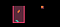12 x 17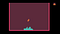50 x 36
`let width = 50;                 // well widthlet height = 36;                // well heightlet square_size = 16;           // square size in pixels`
`let well = new Array(height);   // array holding the entire well`
`// Reset entire well to all 0'sfor (let y = 0; y < width; y++)    well[y] = new Array( height ).fill(0);// Mark bottomfor (let x = 0; x < width; x++)    well[x][height - 1] = 2;// Mark wallsfor (let y = 0; y < height; y++) {    well[y] = 1;    well[width - 1][y] = 1;}`
`// Generate well on the screen by creating HTML elements dynamicallyfor (let y = 0; y < height; y++) {    for (let x = 0; x < width; x++) {        // Create a DIV element dynamically        let square = document.createElement("div");        // Create an intuitive easy to access ID, eg: "square_x5y13"        square.setAttribute("id", "square_x" + x + "y" + y);        // Set some CSS properties for the square        square.style.position = "absolute";        square.style.left = x * square_size + "px";        square.style.top = y * square_size + "px";        square.style.width = square_size + "px";        square.style.height = square_size + "px";        square.style.zIndex = 0;        let block_type = well[x][y];                // Empty space color:        if (block_type == 0) square.style.background = "#082b7f";        // Wall color:        if (block_type == 1) square.style.background = "#841550";        // Bottom wall color:        if (block_type == 2) square.style.background = "#c20c98";        // Add created square to the <BODY> dynamically        document.body.appendChild( square );    }}`

## Tetrominos

The 7 classic patterns consisting of 4 squares are called tetrominos.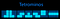The classic set of seven tetrominos.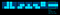The stick is an odd-ball case as it’s the only tetromino that requires a 4x4 grid.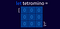Technically, you can use a 2-dimensional array to represent a 9x9 tetromino. But it can also be represented by a 1-dimensional array (pictured here.) This way it’s a bit easier rasterizing it on the well grid.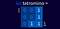One way of defining a tetromino using a 1-dimensional array.
`let A = [0,0,1,         0,0,1,         0,1,1];let B = [1,0,0,         1,0,0,         1,1,0];let C = [0,0,0,         0,1,0,         1,1,1];let D = [0,0,0,         0,1,1,         1,1,0];let E = [0,0,0,         1,1,0,         0,1,1];let F = [1,1,0,         1,1,0,         0,0,0];let G = [0,0,0,         1,1,1,         0,0,0];`
`let tetrominos = [A,B,C,D,E,F,G];`
`let current = [0,0,0, 0,0,0, 0,0,0];let next    = [0,0,0, 0,0,0, 0,0,0];`
`// Generate a random tetromino and return it as 3x3 arrayfunction make_random() {    // 1.) Select random tetromino from tetrominos array by index    let index = Math.floor((Math.random() * tetrominos.length));    // 2.) Copy it into current array (avoid reference assignment)    return [...tetrominos[ index ]];}`
`current = make_random();next = make_random();`

## Keyboard Controls

Here is the keyboard controls source code:

`// Keyboard inputdocument.addEventListener("keydown", (e) => {    let key_code = e.keyCode;    // Erase the teetromino    erase();    // Left    if (key_code == 37) {        if (will_collide(dir.LEFT)) {            reset();        } else position.x -= 1    }    // Right    if (key_code == 39) {        if (will_collide(dir.RIGHT)) {            reset();        } else position.x += 1    }    // Down    if (key_code == 40) {        if (will_collide(dir.DOWN)) {            reset();        } else position.y += 1    }    if (key_code == 38) { position.y -= 1 }    // Rotate    if (key_code == 90) { rotate_left() }    if (key_code == 88) { rotate_right(); }    // Draw the current tetromino    draw();});`

## Falling Animation

The game loop consists of erase, fall and draw functions.

`// Game-loop AnimationsetInterval(() => {    // Erase the current tetromino block from the well    erase();    // Progress the tetromino by 1 square down    fall();    // Draw the tetromino at its new fallen position    draw();}, 15);`

## Collision Detection

There are two types of collisions in Tetris. With walls and with fallen bricks.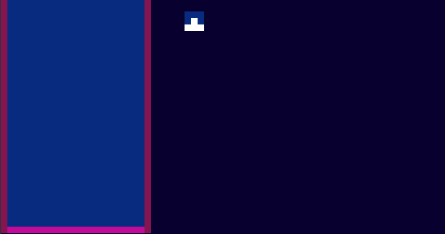Avoid writing collision detection in “real time.” You need to figure out if the current block will collide at a future time IF it is moved in the direction it is moving on the next frame, not on the current frame. And if there is a future collision, prevent any further movement and “paste” the brick into the well as a solid block (the latter is not shown on this animation, it will be explained in one of the following sections.)
`// Left arrow key is pressedif (key_code == 37) {    // Will tetromino collide with walls or if it is moved left?    if (will_collide( dir.LEFT ))        reset();    else       // Tetromino will not collide, move to that position        position.x -= 1;}`

## reset()

The reset function is more of a helper function. It calls paste(), clear_row(), make_random(), update_next() and erases the fog of darkness.

`function reset() {    paste();                // paste current tetromino onto the well    clear_row();            // clear rows if any    current = [...next];    // swap current and next tetromino    next = make_random();   // generate next tetromino    update_next();          // Update "next" box    // reset current position to top and middle of the well    position.x = parseInt(width/2) - 1;    position.y = -3;    reset_fog();             // clear the fog of darkness}`

## paste()

`// "paste" current block onto the wellfunction paste() {    let index = 0;    // Prevent pasting blocks that fall outside of the well:    if (position.x >= 0 && position.x <= width - 1) {        if (position.y >= -3 && position.y <= height - 1) {            // Iterate over the 3x3 block of tetromino:            for (let y = position.y; y < position.y + 3; y++) {                for (let x = position.x; x < position.x + 3;                     x++, index++) {                    // If tetromino is solid at that square                    if (current[index] == 1) {                        let id = "square_x" + x + "y" + y;                        let sq = document.getElementById(id);                        if (sq) {                            well[x][y] = 3;                            sq.style.backgroundColor = color.solid;                        }                    }                }            }        }    }}`

## erase()

It’s the same as paste() only it sets the currently falling tetromino to all 0’s, effectively erasing it from the well array (before animating it to next position.)

`function erase() {    let index = 0;    if (position.x >= 0 && position.x <= width - 1) {        if (position.y >= -3 && position.y <= height - 1) {            for (let y = position.y; y < position.y + 3; y++) {                for (let x = position.x; x < position.x + 3;                     x++, index++) {                    if (current[index] == 1) {                        let id = "square_x" + x + "y" + y;                        let square = document.getElementById(id);                        if (square) {                            if (true) { // well[x] && well[y]                                well[x][y] = 0;                                if (x == 0 || x == width - 1 ) { }                                else {                                    square.style.backgroundColor                                    = color.background;                                }                            }                        }                    }                }            }        }    }}`

## Pasting The Fallen Block Into The Well

Once a block is considered “fallen” it is physically pasted into the well array.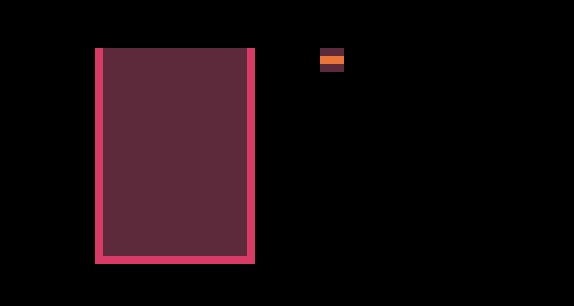Once a brick collides with walls or other bricks, it gets “pasted” into the well and marked as solid.

## Row Breaking Algorithm

This is the most complex piece of code when it comes to Tetris. This algorithm will check if 1) there are any rows to clear 2) rebuild the well again without the complete rows to cancel them out and create block collapsing illusion.

`// Check if a row needs to be clearedfunction clear_row() {    // Placeholder for new rows    let placeholder = [];    // How many rows cleared?    let rows_cleared = 0;    // Scan the well one row at a time and capture any    // non-filled rows in placeholder    // (except the last row)    for (let y = 0; y < height - 1; y++) {        let start = y * width;        let scanned = scan_row(y);        let total = scanned;        let row_data = scanned;        // Skip all horizontal rows that are completely filled        if (total != width) {            // Memorize only uncleared rows            let len = placeholder.length;            placeholder[len] = row_data;        } else {            start_highlight(y);            rows_cleared++;        }    }    // If at least one row was cleared, update the well    if (rows_cleared > 0) {        // Clear the well, except last row (well's bottom)        for (let y = 0; y < height - 1; y++) {            // Clear all except walls ( and [width - 1])            for (let x = 1; x < width - 1; x++) {                well[x][y] = 0;                // Paint empty square                let square =                document.getElementById("square_x" + x + "y" + y);                if (square)                    square.style.backgroundColor = color.background;            }        }        // Paste captured placeholder rows onto the well        // but from bottom up        let r = height - 2;        for (let i = placeholder.length - 1; i > 0; i--) {            let row = placeholder[i];            for (let x = 0; x < width; x++) {                if (row[x] != 0) {                    well[x][r] = 3;                    if (x != 0 && x != width - 1) {                        let square =                        document.getElementById("square_x"+x+"y"+r);                    if (square)                        square.style.backgroundColor = color.solid;                    }                }            }            r--;        }    }}`

## Tetris In The Dark (Included in source code!)

Strategy video games have something called for of war. It covers an unexplored area of terrain with blackness.

## Adding Light

To create a light spot, first I simply created a secondary grid sharing the same dimensions as the well and used it as an overlay. By default all DIV squares on that grid were set to black color and opacity of 1.

`// light positionlet light = { x: 0, y: 0 };// lightspot datalet light_mask = [    0,0,0,0,0,0,0,0,0,0,0,0,0,0,    0,0,0,0,0,0,4,4,0,0,0,0,0,0,    0,0,0,2,3,4,5,5,4,3,2,0,0,0,    0,0,2,3,4,5,6,6,5,4,3,2,0,0,    0,2,3,4,5,6,7,7,6,5,4,3,2,0,    0,3,4,5,6,7,8,8,7,6,5,4,3,0,    0,4,5,6,7,8,9,9,8,7,6,5,4,0,    0,4,5,6,7,8,9,9,8,7,6,5,4,0,    0,3,4,5,6,7,8,8,7,6,5,4,3,0,    0,2,3,4,5,6,7,7,6,5,4,3,2,0,    0,0,2,3,4,5,6,6,5,4,3,2,0,0,    0,0,0,2,3,4,5,5,4,3,2,0,0,0,    0,0,0,0,0,0,4,4,0,0,0,0,0,0,    0,0,0,0,0,0,0,0,0,0,0,0,0,0,];`
`function draw_light() {    let index = 0;    for (let y = 0; y < 14; y++) {        for (let x = 0; x < 14; x++, index++) {            let posx = x + position.x;            let posy = y + position.y;            let id = "fog_x" + (posx - 6) + "y" + (posy - 6);            let square = document.getElementById(id);            if (square) {                let type = light_mask[index];                if (type == 9) square.style.opacity = '0';                if (type == 8) square.style.opacity = '0.1';                if (type == 7) square.style.opacity = '0.2';                if (type == 6) square.style.opacity = '0.3';                if (type == 5) square.style.opacity = '0.4';                if (type == 4) square.style.opacity = '0.5';                if (type == 3) square.style.opacity = '0.7';                if (type == 2) square.style.opacity = '0.8';                if (type == 1) square.style.opacity = '0.9';                if (type == 0) square.style.opacity = '1.0';            }        }    }}`

# Breaking Row Animation

The row-breaking animation is done separately from everything else. It’s just a list of long horizontal DIV elements at every height level of the well.

# Final Results

When working with the same subject for a long period of time you tend to get a bit bored and an impulse to innovate awakens.

Issues. Every webdev has them. Published author of CSS Visual Dictionary https://amzn.to/2JMWQP3 few others…

## More from JavaScript Teacher

Issues. Every webdev has them. Published author of CSS Visual Dictionary https://amzn.to/2JMWQP3 few others…